# Kerala SSLC Maths Model Question Paper 1 English Medium

Students can Download Kerala SSLC Maths Model Question Paper 1 English Medium, Kerala SSLC Maths Model Question Papers helps you to revise the complete Kerala State Syllabus and score more marks in your examinations.

## Kerala SSLC Maths Model Question Paper 1 English Medium

Time: 2½ Hours
Total Score: 80 Marks

Instructions

• Give explanations wherever necessary.
• First 15 minutes is Cool-off time. You may use the time to read the questions and plan your answers.
• No need to simplify irrationals like √2, √3, π etc., using approximations unless you are asked to do so.

Answer any three questions from 1 to 4. Each question carries 2 scores. (3 × 2 = 6)

Wandoor Ganitham Sslc Model Question Paper Question 1.
Consider the arithmetic sequence 13, 23, 33, …..
a) What is its common difference?
b) What is the first three-digit term of this sequence?
(a) 10
(b) 103

Wandoor Ganitham Model Question Paper Question 2.
In the figure, O is the centre of the circle ∠A = 60°.a) ∠BOD = __________
b) ∠C = _________
(a) ∠BOD = 120°
(b) ∠C = 120°

Wandoor Ganitham Answer Key Polynomials Question 3.
If x – 1 is a factor of the polynomial 5x3 – 4x2 + x – k, what number is k?
P(x) = 5x3 – 4x2 + x – k
P(1) = 5 × 13 – 4 × 12 + 1 – k = 0
5 – 4 + 1 – k = 0
k = 2

Wandoor Ganitham Question Paper Question 4.
A circle is drawn with the origin as centre. It passes through the point (3, 3).
a) What is the radius of the circle?
b) Write the coordinates of a point where the circle meets the x-axis.
(b) (-3√2, 0)Answer any five questions from 5 to 11. Each question carries 3 scores. (5 × 3 = 15)

Wandoor Ganitham Answer Key 2021 Question 5.
a) If a square is inscribed in a circle of diameter 4cm, what will be the length of a side of the square?
b) What is the base edge of a square pyramid of maximum size that can be carved out from a hemisphere of radius 5 cm?
(a) Base edge of the square pyramid = 2√2 cm
(b) Base edge = $$\frac{10}{\sqrt{2}}=\frac{5 \times 2}{\sqrt{2}}$$
\begin{aligned} &=\frac{5 \times \sqrt{2} \times \sqrt{2}}{\sqrt{2}}\\ &=5 \sqrt{2} \mathrm{cm} \end{aligned}

Wandoor Ganitham Model Question Paper 2021 Question 6.
Draw the co-ordinate axes and mark the point (4, 0). Draw an isosceles right angled triangle with this point as one of its vertices.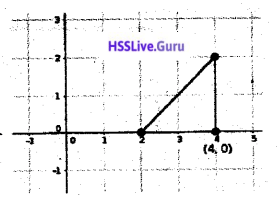Wandoor Ganitham Answer Key Arithmetic Sequence Question 7.
Draw a circle of radius 3.5 centimetres. Draw a triangle of angles 50°, 60°, 70°with its vertices as points on the circle.Wandoor Ganitham Model Question Paper Answer Key Question 8.
The algebraic form of an arithmetic sequence is 5n + 5
(a) What is its first term?
(b) What is the difference pf its 10th and 20th terms?
(c) Can the difference between any two terms of this sequence be 368? Justify.
(a) x1 = 5 × 1 + 4 = 9
(b) Difference: x20 – x10 = 10d = 10 × 5 = 50
(c) The difference between any two terms of an arjthmetic sequence is divisible by the common difference.
368 is not divisible by 5, the common difference that is, 368 is not the difference btween two terms.

Sslc Model Question Paper Maths English Medium Question 9.
The length of a rectangle is 6 centrimetres more than its breadth. Its area is 1216 square centimetres. Find its length.
Length = x + 6
x(x + 6) = 1216
⇒ x2 + 6x = 1216
⇒ x2 + 6x + 9 = 1216 + 9 = 1225
⇒ (x + 3)2 = 1225
⇒ x + 3 = √1225 = ±35
⇒ x + 3 = 35 or x + 3 = -35
⇒ x = 35 – 3 = 32 or x = -35 – 3 = -38
Length = 32 + 6 = 38

Sslc Maths Question Paper 2020 Kerala English Medium Question 10.
In the figure, C, D are points on the circle AD is a diameter of the circle. ∠C = 30°, AB = 4 centrimetres.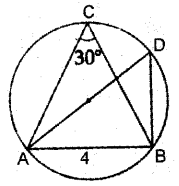a) ∠D = ________
b) ∠ABD = _________
c) What is the length of the diameter?
(a) ∠D = 30° (Angle in the same arc)
(b) ∠ABD = 90° (Angle in the semicircle is 90°)
(c) 8 cm

Sslc Maths Model Question Paper English Medium Question 11.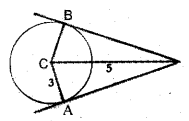In the figure C is the centre of the circle, PA and PB are tangents. PC = 5 centimetres and radius of the circle is 3 centimetres.
a) Find the length of PA
b) What is the area of the quadrilateral PACB?
(a) PA = 4 cm
(b) Area of PAC = $$\frac { 1 }{ 2 }$$ × 4 × 3 = 6 cm2
Area of PACB = 2 × 6 = 12 cm2

Answer any 7 questions from 12 to 21. Each question carries 4 scores. (7 × 4 = 28)

Wandoor Ganitham Sslc Model Question Paper Answer Key Question 12.
The coordinates of two opposite vertices of a rect-angle are (7, 8) and (1, 3).
a) Without drawing coordinate axes, mark these points as the vertices of a rectangle with left-right, top-bottom positions correct.
b) Find the co-ordinates of other two vertices.
c) What is the length of its diagonals?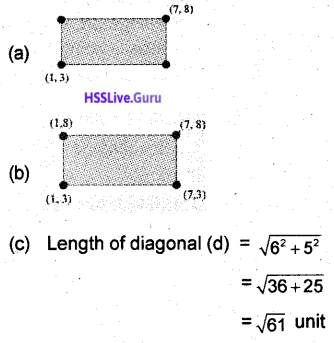Wandoor Ganitham Sslc Model Answer Key 2021 Question 13.
Consider the polynomial P(x) = ax3 – x2 – bx – 1
a) Find P(1).
b) What is the relation between a and b if x – 1 is a factor of P(x)?
c) What is the relation between a and b if x + 1 is a factor of P(x)?
d) Will, P(x) have both (x + 1) and (x – 1) as factors for any numbers a and b? Justify.
P(x) = ax3 – x2 – bx – 1
(a) P(1) = a × 13 – 12 – b × 1 – 1 = a – 1 – b – 1 = a – b – 2
(b) If (x – 1) is a factor, P(1) = 0
ie. a – b – 2 = 0
ie. a – b = 2
(c) If x + 1 is a factor, P(-1) = 0
ie. a (-1)3 – (-1)2 – b(-1) – 1 =0
ie. -a – 1 + b – 1 = 0
ie. b – a = 2
(d) It is not possible to find a and b such that a – b = b – a = 2
Therefore we cannot find a and b.

Hss Live Guru Model Question Paper Question 14.
The radius and height of a cone are 12 centimetres and 6 centimetres respectively.
a) What is its volume?
b) If this cone is cut parallel to its base, along the midpoint of its height, what is the radius of the small cone obtained?
c) What is the volume of the small cone?
d) Find the ratio of the volumes of the small cone and the first cone.
r = 12 cm, h = 6 cm
(a) Volume = $$\frac { 1 }{ 3 }$$πr2h
= $$\frac { 1 }{ 3 }$$ × π × 122 × 6 = 288π cm3
(b) R = 12 cm, H = 6 cm, h = 3 cm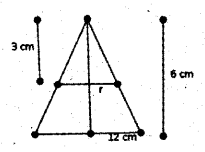\begin{aligned} &\frac{r}{R}=\frac{h}{H}\\ &\frac{r}{12}=3 \end{aligned}
r = 6 cm
(c) Volume = $$\frac { 1 }{ 3 }$$πr2h
= $$\frac { 1 }{ 3 }$$ × π × 62 × 3 = 36π cm3
(d) Ratio = 36π : 288π = 1 : 8

Wandoor Ganitham Sslc Unit Test 2021 Answer Key Question 15.
In the figure ABCD is a parallelogram. ∠E = 90°. A(3, 5) B(8, 5) are two vertices BE = 3 units, CE = 4 units.a) Write the coordinates of C.
b) What are the coordinates of D?
c) Find the coordinates of meeting point of the di-agonals of the parallelogram.
(a) B(8, 5), BE = 2
E(11, 5), C (11, 9)
(b) D (6, 9)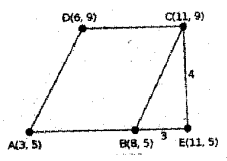(c) The point of intersection of the diagonals is the midpoint of a diagonal.
The mid point of AC is $$=\left(\frac{11+3}{2}, \frac{9+5}{2}\right)$$ = (7, 7)

Wandoor Ganitham Pre Model Answer Key Question 16.
Draw a circle of radius 3 centrimetres. mark a point 7 centimetres away from its centre. Draw the tan¬gents to the circle from that point.Hsslive Guru Question Paper Question 17.
In triangle PQR, ∠Q = 90°, ∠R = x°. Lengths of the sides PQ, QR and PR are a, b, c respectively.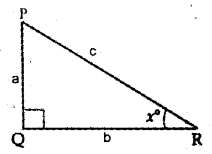a) Which among the following is tan x°?
$$\left(\frac{a}{c}, \frac{b}{a}, \frac{a}{b}, \frac{b}{c}\right)$$
b) Similarly write sin x° and cos x° from this triangle.
c) Prove that $$\frac{\sin x^{0}}{\cos x^{0}}=\tan x^{0}$$Question 18.
Consider two fractions having numerator 1. The denominator of one fraction is 2 more than the denominator of the other. Sum of these fractions is $$\frac{5}{12}$$.
a) Write the above fact as an algebraic equation.
b) Find the fractions.
Let x be the denominator of the first fraction.
The denominator of the other fraction is x + 2Question 19.
A bag contains some red and green balls. If we take a ball from it, without looking, the probability of getting a red ball is $$\frac{1}{4}$$
a) What is the total number of balls, if there are 8 red balls?
b) What is the probability that a ball taken is green?
c) Find the sum of both the probabilities.
d) From a box containing some red balls and some blue balls th& probability of getting a red ball is $$\frac{a}{b}$$. What is the probability of getting a blue ball?Question 20.
In the figure, ∠P = 90°. Sides of triangle APC are extended to B and D.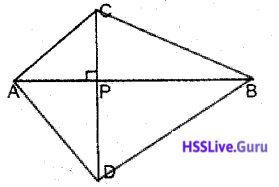a) If a circle is drawn with AC as its diameter, where will be the position of P with respect to that circle?
b) What about the position of P, if the circle is drawn with AD as diameter?
c) Prove that, the circles drawn with the sides of a quadrilateral with perpendicular diagonals, will meet at a common point.
(a) On the circle
(b) On the circle
(c) Since AB and CD are perpendicular to each other, the circle with diameter AB, diameter AD, diameters DB and diameter GB passes through P.

Question 21.
a) Write the sequence of odd numbers greater than 1.
b) What is the algebraic form of this sequence?
c) What is the algebraic form of the arithmetic sequence $$\frac{3}{6}, \frac{5}{6}, \frac{7}{6} \ldots .$$
d) Prove that this sequence does not contain any natural number.
(a) 3, 5, 7, …
(b) xn = 2n + 1
(c) xn = $$\frac{2 n+1}{6}$$
(d) To get integer terms, the numerator should be a multiple of 6.
2n + 1 is odd. It is not a multiple of 6 for carry n.
The sequence does, not contain integers.

Answer any five questions from 22 to 28. Each question carries 5 score. (5 × 5 = 25)

Question 22.
The table below shows the students of a class sorted according to their scores in a test

 Score Number of Students 0 – 10 5 12 – 20 9 20 – 30 10 30 – 40 9 40 – 50 8

a) If the students are arranged in the increasing order of their scores, the score of the student at what position is taken as the median?
b) What may be assumed as the score of the 15th student in the arrangement?
c) Find the median score.

 Score No. of children Less than 10 5 Less than 20 14 Less than 30 24 Less than 40 33 Up to 50 41

(a) n = 41 (odd number)
$$\frac{41+1}{2}=\frac{42}{2}$$ = 21st score comes in the middle.
It is in between 20 and 30
(b) When 10 scores we equally divided among 10 children, each one’s share is $$\frac { 10 }{ 10 }$$ = 1
Score of 15th child = 20 + $$\frac { 1 }{ 2 }$$ = 20.5
(c) Consider the arithmetic sequence with first term 20.5 and common difference 1
7th term will be the median Median = 20.5 + 6 × 1 = 20.5 + 6 = 26.5

Question 23.
A solid is made by fixing a hemisphere of same radius on the flat face of a cone. The height of the cone is 12 centimetres and its slant height is 13 centimetres.a) What is the radius of the cone?
b) What is the curved surface area of the hemisphere?
c) What is the total surface area of the solid?
l = 13 cm, h = 12 cm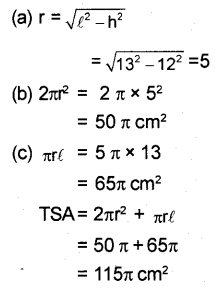Question 24.
Draw a triangle of sides 5 dentimetres, 6 centimetres and 7 centimetres. Draw its incircle.
Draw triangle, Draw angle bisectors. They meet at a point. That point will be the incenter.Question 25.
A boy is standing between two buildings of equal height. The boy and the buildings are in a straight line. He sees the tops of those buildings at elevations of 45° and 30°. The nearest building is 20 metres away from him.
a) Drawa rough figure.
b) Find the height of the buildings and distance between the buildings.
(a)(b) BC = 20m, ∠CBD = 45°, CD = 20 m
Height of the building = 20 m
AE = 20 m
ie. AB = 20√3 m
Distance between the building 20 + 20√3 = 20(1 + √3)m

Question 26.
In the figure chords AB and CD intersect at P. PA = 8 centrimetres, PB = 6 centimetres, PC = 4 centimetres, BC = 4 centimetres.a) Which angle is equal to ∠A?
b) Write one more pair of equal angles.
c) Find the length of PD.
d) What is the length of AD?
(a) ∠A = ∠C
(b) ∠D = ∠B
(c) PD × PC = PA × PB
PD × 4 = 8 × 6
PD = 12 cm
(d) ΔAPD , ΔCPB are similar
\begin{aligned} &\frac{A P}{P C}=\frac{P D}{P B}=\frac{A D}{B C}\\ &\frac{8}{4}=\frac{A D}{4} \end{aligned}

Question 27.
The sum of first 9 terms of an arithmetic sequence is 45 and the sum of first 18 terms is 171.
a) What is the sum of its 10th to 18th terms?
b) What is tis 5th term?
c) Find its 14th term.
d) Find the sum of 5th to 14th terms.
S9 = 45, S18 = 171
(a) If Sn denotes the sum of first n terms = S18 – S9 = 171 – 45 = 126
(b) $$x_{5}=\frac{S_{9}}{9}=\frac{45}{9}=5$$
(c) $$x_{14}=\frac{126}{9}=14$$
(d) Sum of 5th to 14th terms = $$\frac { 10 }{ 2 }$$ (X5 + X14)
= 5(5 + 14)
= 5 × 19
= 95

Question 28.
In the figure ABCD is a square. The axes are drawn throgh the midpoints of its sides. The length of its side is 6 units.a) Write the coordinates of its vertices.
b) Write the equation of its diagonal BD.
(a) A (-3, -3) B(3, -3) C (3, 3) D (-3, 3)
(b) Slope of BD = $$\frac{Y_{2}-Y_{1}}{X_{2}-X_{1}}=\frac{3–3}{-3-3}=\frac{6}{-6}=-1$$
Let (x, y) be a point on BD
$$\frac{y-3}{x-3}=-1$$
y + 3 = -1(x – 3)
y + 3 = -x + 3
x + y = 0

Read the following. Understand the Mathematical Idea expressed in it and answer the questions that follow. (6 × 1 = 6)

Question 29.
Consider the number 2751. The sum of its digits is 2 + 7 + 5 + 1 = 15. Adding the digits of 15 we get 1 + 5 = 6. This number 6 is called the ‘digital root’ of the number 2751. That is, to find the digital root of a number, find the sum of its digits (Dont forget to find the sum of the digits again if the first sum has more than one digit)
Let us see one more example.
The sum of the digits of the number 679412 is 6 + 7 + 9 + 4 + 1 + 2 = 29
Sum of digits of 29 = 2 + 9 = 11
Sum of digits of 11 = 1 + 1 = 2
Therefore the digital root of 679412 is 2.
Digital roots have an interesting property.
To see this, consider the product 43 × 27 = 1161.
The digital roots of the numbers 43 and 27 are 4 + 3 = 7 and 2 + 7 = 9.
Product of the digital roots = 7 × 9 = 63.
Digita root of 63 = 6 + 3 = 9.
The digital root of 1161 is also 9 (1 + 1 + 6 + 1 = 9)
That is the digital root of 1161 = The digital root fo 63, where 63 is the product of the digital roots of 43 and 27.
This property is true for all other natural numbers.
a) What is the digital root of 345?
b) What is the digital root of 927?
c) What is the digital root fo 345 × 927?
d) The digital root of the number 63 □ 5 is 8 (□ represents a missing digit). Find the missing digit.
e) 121 × 92 = 11□32. Find the missing digit.
f) If the digital root of a is 5 and the digital root of b is 2. Then what is the digital root of ab?
(a) 3 + 4 + 5 = 12 = 1 + 2 = 3
(b) 9 + 2 + 7 = 18 = 1 + 8 = 9
(c) 9 × 3 = 27 = 2 + 7 = 9
(d) 6 + 3 + x + 5 = 8
14 + x = 8
1 + 4 + x = 8
5 + x = 8
x = 8 – 5 = 3
(e) (1 + 2 + 1) (9 + 2) = 1 + 1 + X + 3 + 2
4(1 + 1) = 7 + x
4 × 2 = 7 + x
8 = 7 + x
x = 1
(f) Digital root f a × b = 5 × digital root of 2
5 × 2 = 10
Digital root of 10 is 1.